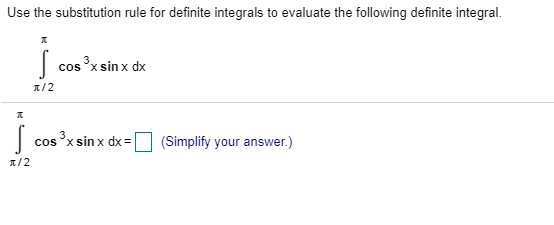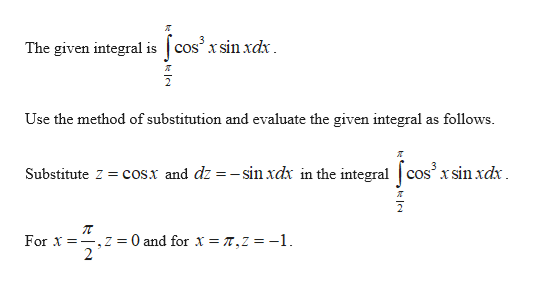Use the substitution rule for definite integrals to evaluate the following definite integral.Jcos x sinx dx/23cos xsin x dx(Simplify your answer.)/2

Questionhelp_outlineImage TranscriptioncloseUse the substitution rule for definite integrals to evaluate the following definite integral. J cos x sinx dx /2 3 cos xsin x dx (Simplify your answer.) /2 fullscreen
Step 1help_outlineImage TranscriptioncloseThe given integral iscos' x sinxdx Use the method of substitution and evaluate the given integral as follows Substitute Z cos.x and dz =-sinxdx in the integral |cos' x sin xdx 0 and for x T, Z = -1. For -.Z = 2 fullscreen

Want to see the full answer?

See Solution

Want to see this answer and more?

Our solutions are written by experts, many with advanced degrees, and available 24/7

See Solution
Tagged in

Integration